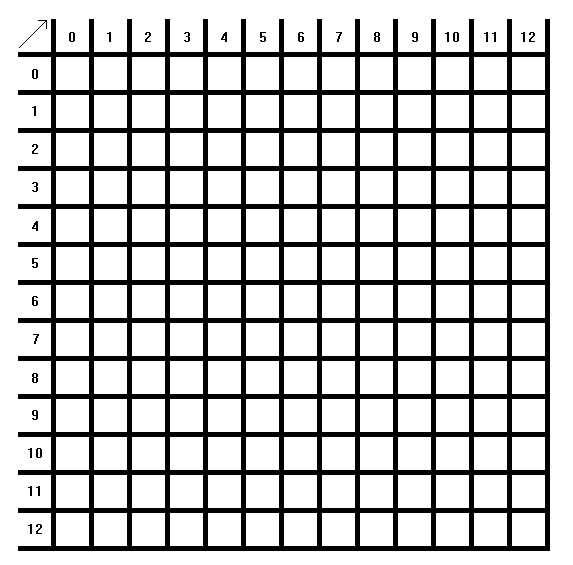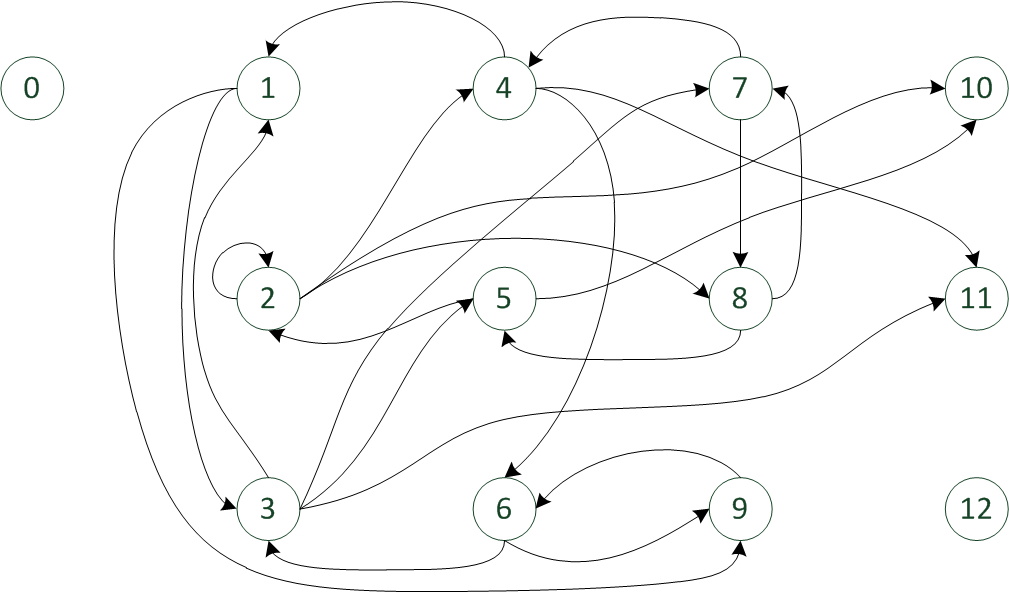# LoveGraph

Show your love with a graph !
To do so, simply print the matrix and the directed graph (nodes/edges), and give the instructions.
(If they are new to the algorithms and computer science courses, give them an example with a directed edge between two nodes, for example, write "4 -> 6" and color the corresponding square)

Here is the empty matrix :Here is the directed graph :Here is the list of edges between nodes :

Text

Here is the current "answer" :